# 概述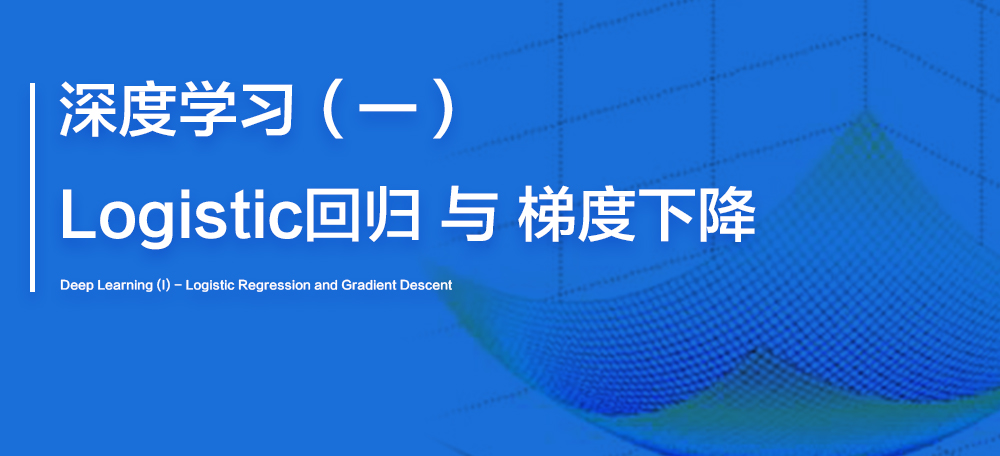# 1.0 Logistic 回归概述

Logistic 回归是一种广义上的线性回归，也是一个用在监督学习当中的学习算法，一般用于输出值为0或1，即”是”或”不是”的问题当中，这就是二元分类问题。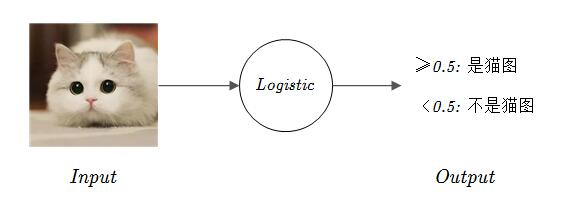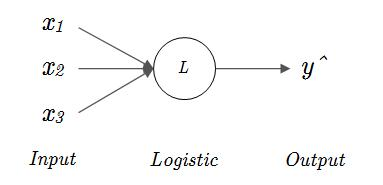# 1.1 sigmoid 激活函数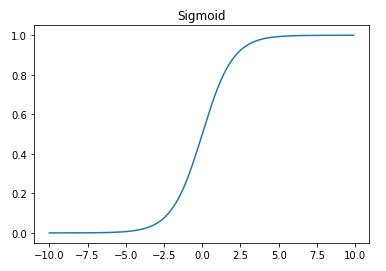• 当 $z$ 越来越小时，$e^{-z}$ 就会越来越大，对应的表达式的分母就会越来越大，使得 $\sigma(z)$ 逐渐趋于0；
• 当 $z$ 越来越大时，$e^{-z}$ 就会越来越小并趋近于0，对应的表达式的分母就会越来越趋近于1，使得 $\sigma(z)$ 逐渐趋于1。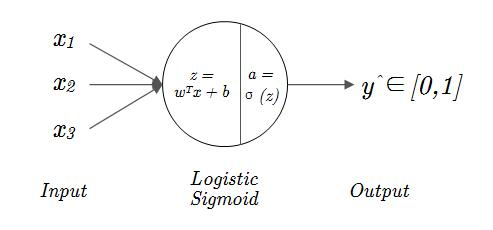# 1.2 Logistic 回归损失函数

## Loss Function

1.当正确输出 $y=0$ 时，表达式变成了公式(8)，为了使得 $L(\hat{y},y)$ 尽可能小，$log(1-\hat{y})$ 就要尽可能大，也就是 $1-\hat{y}$ 要尽可能大，最终结果是 $\hat{y}$ 要尽可能小，而 $\hat{y} \in [0,1]$，因此 $\hat{y}$ 需要尽可能接近于0，也就是正确输出 $y$。

2.当正确输出 $y=1$ 时，表达式变成了公式(9)，为了使得 $L(\hat{y},y)$ 尽可能小，$log(\hat{y})$ 就要尽可能大，最终结果是 $\hat{y}$ 要尽可能大，而 $\hat{y} \in [0,1]$，因此 $\hat{y}$ 需要尽可能接近于1，也就是正确输出 $y$。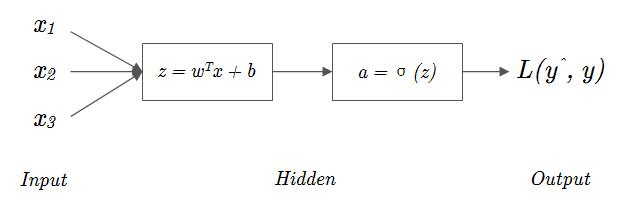## Cost Function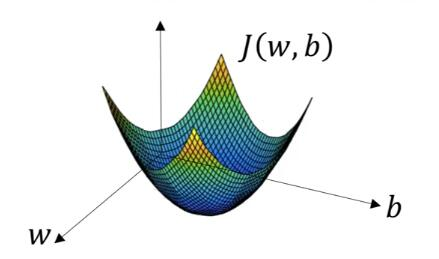# 1.3 梯度下降法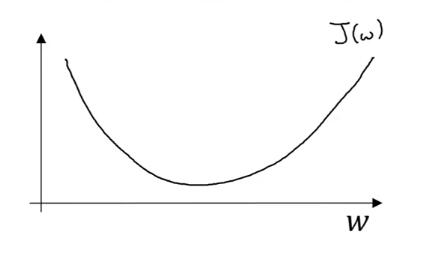# 1.4 Logistic 回归中的梯度下降法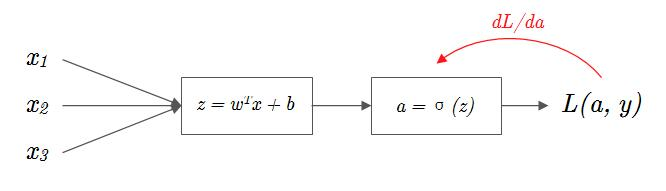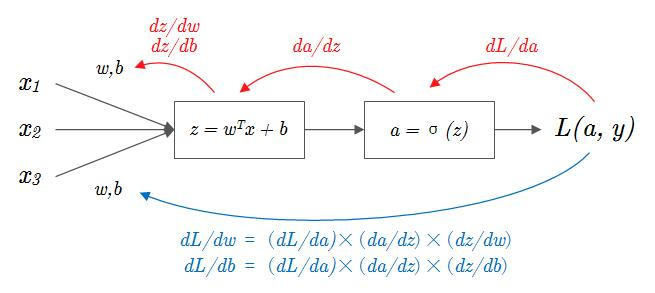# 1.5 本篇小结

1. 二元分类问题中的 Logistic 回归是怎样的流程？（图1-9
2. 为什么要引入 Sigmoid 激活函数？（将 $z=w^Tx+b$ 映射为区间 $[0,1]$ 上的一个概率
3. Logistic 回归中使用什么作为损失函数？（Loss Function / Cost Function
4. 梯度下降法的“下降”核心可以分为哪两部份？（学习率 $\alpha$ 与 梯度(斜率)
5. Logistic 回归中参数 $w$ 与 $b$ 的迭代公式是？（公式(14)(15) 或 公式(26)(27)

﻿
0%